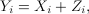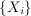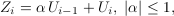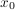# MA(1) feedback capacity

### Feedback capacity of the first-order moving average Gaussian channel

Young-Han Kim

Despite numerous bounds and partial results, the feedback capacity of the stationary nonwhite Gaussian additive noise channel has been open, even for the simplest cases such as the first-order autoregressive Gaussian channel studied by Butman, Tiernan and Schalkwijk, Wolfowitz, Ozarow, and more recently, Yang, Kavcic, and Tatikonda. Here we consider another simple special case of the stationary first-order moving average additive Gaussian noise channel and find the feedback capacity in closed form. Specifically, the channel is given bywhere the inputsatisfies a power constraint and the noiseis a first-order moving average Gaussian process defined bywith white Gaussian innovationsWe show that the feedback capacity of this channel iswhereis the unique positive root of the equationandis the ratio of the average input power per transmission to the variance of the noise innovation. The optimal coding scheme parallels the simple linear signaling scheme by Schalkwijk and Kailath for the additive white Gaussian noise channel — the transmitter sends a real-valued information-bearing signal at the beginning of communication and subsequently refines the receiver's knowledge by processing the feedback noise signal through a linear stationary first-order autoregressive filter. The resulting error probability of the maximum likelihood decoding decays doubly-exponentially in the duration of the communication. Refreshingly, this feedback capacity of the first-order moving average Gaussian channel is very similar in form to the best known achievable rate for the first-order {autoregressive} Gaussian noise channel given by Butman.